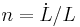# User:Issa Rice

(diff) ← Older revision | Latest revision (diff) | Newer revision → (diff)
Jump to: navigation, search

test >:(

## Questions

• How do we measure growth? How do we know what's growing?
• How is GDP calculated?
• What choices/judgment calls are made in the calculation?
• What's up with all these price indices/PPP formulas/aggregation methods?
• Basket construction
• Validation
• Going out and measuring things (surveys? taxation? some old dutch guy at a port kept import records?)
• Can we trust these GDP calculations?

## Growth models

Model name Endogenous variables Exogenous variables Constants Year introduced
Harrod–Domar 1939
Solow–Swan 1956
AK
Leontief input–output
Feldman–Mahalanobis
Becker–Barro 1981, 1989
Ramsey–Cass–Koopmans
Romer 1990?
Schumpeterian
Product-variety
Semi-endogenous (Jones)
Production function name Models that use this production function
Cobb–Douglas Solow–Swan
Leontief

Probably for each model, a table like this:

Name Variable Unit Rival input? Endogenous? Notes
Output Y Yes
Physical capital (capital stock) K Yes In Solow–Swan Physical capital includes things like machines, computers, buildings, etc.
Labor L Yes Exogenous in Solow–Swan
Technology (knowledge) A, T No Not in Solow–Swan
Consumption C
Investment I
Amount saved S
Growth of X$g_X = \dot{X}/X = \frac{\frac{\partial X}{\partial t}}{X}$
Population growth$n = \dot L / L$
Depreciation (rate?) δ, d, D
Capital per worker k = K/L In Solow–Swan
Fraction saved s
Output per worker y = Y/L Yes
Time t
Production function F
α